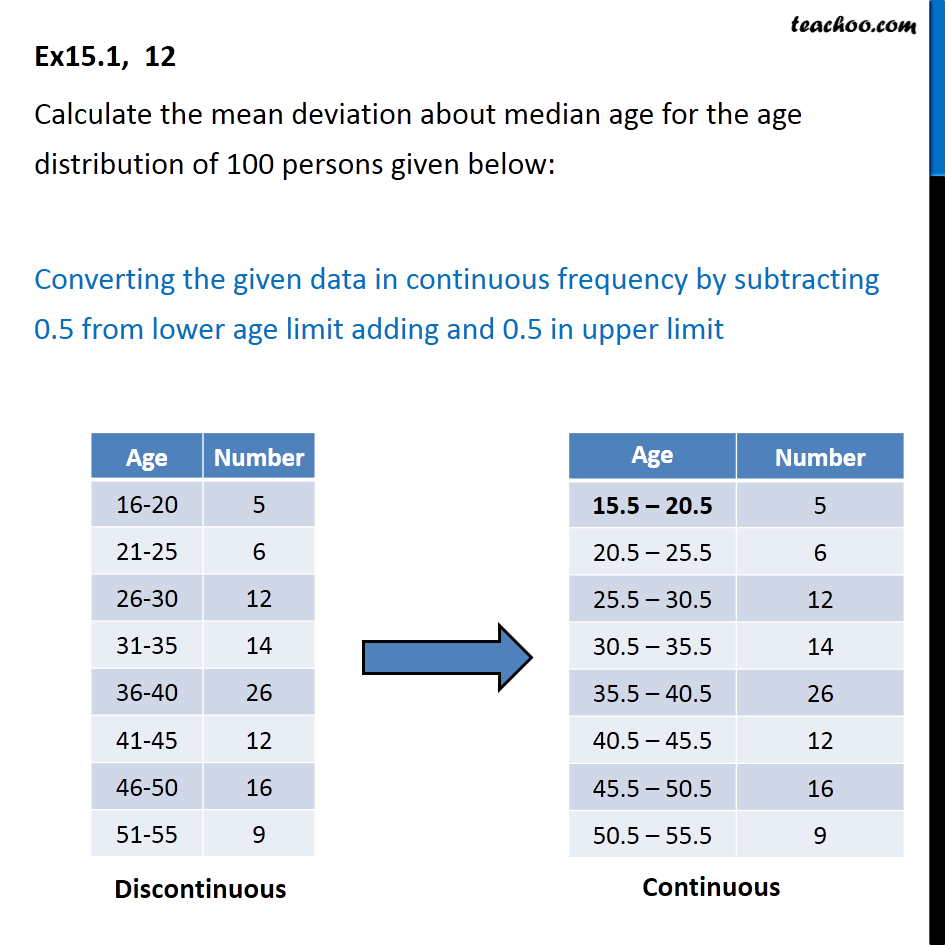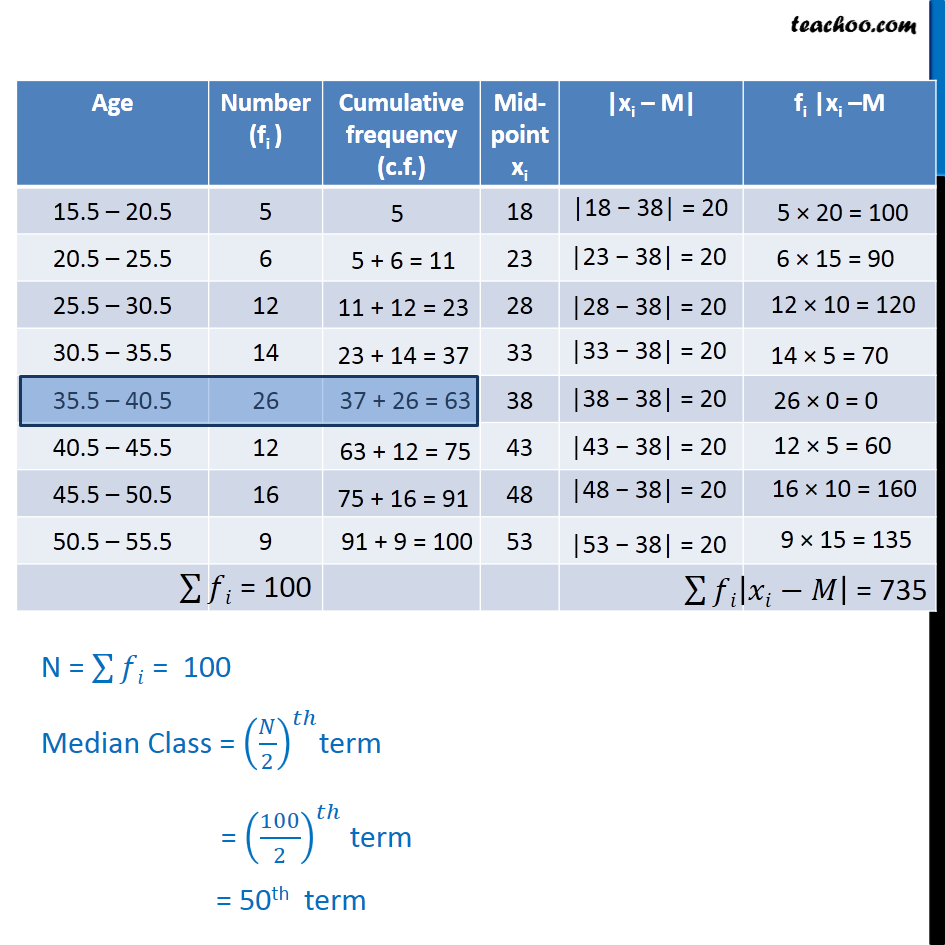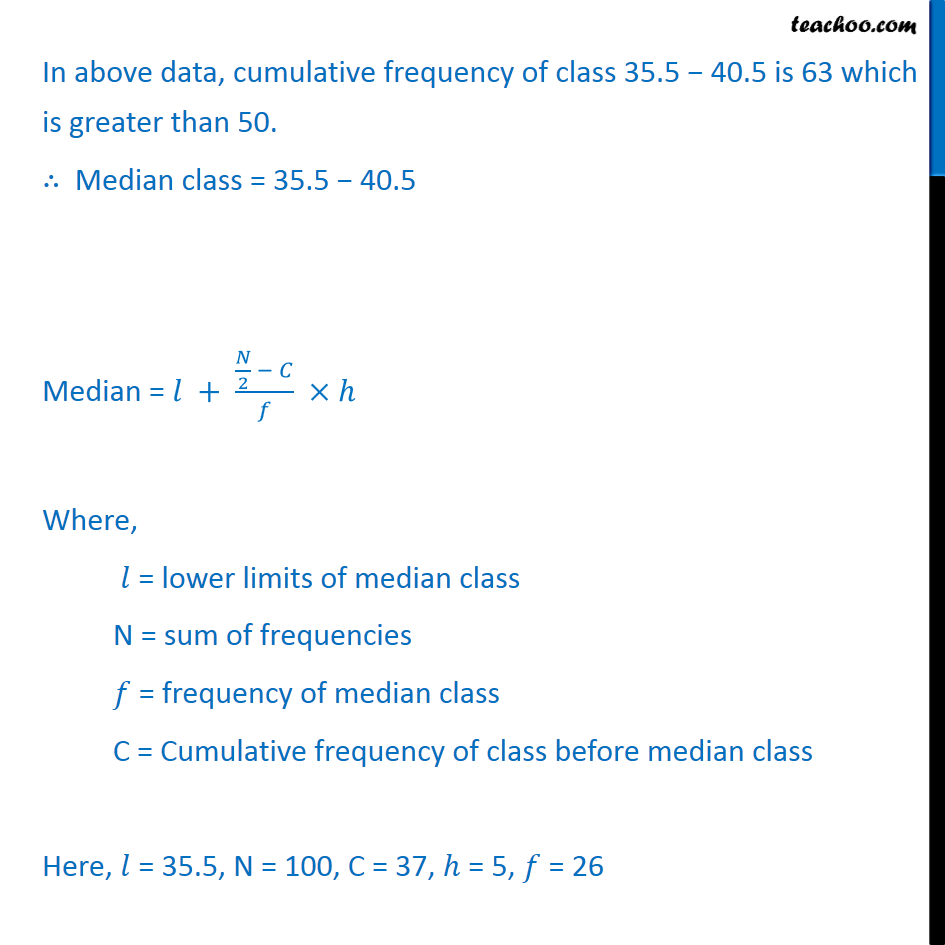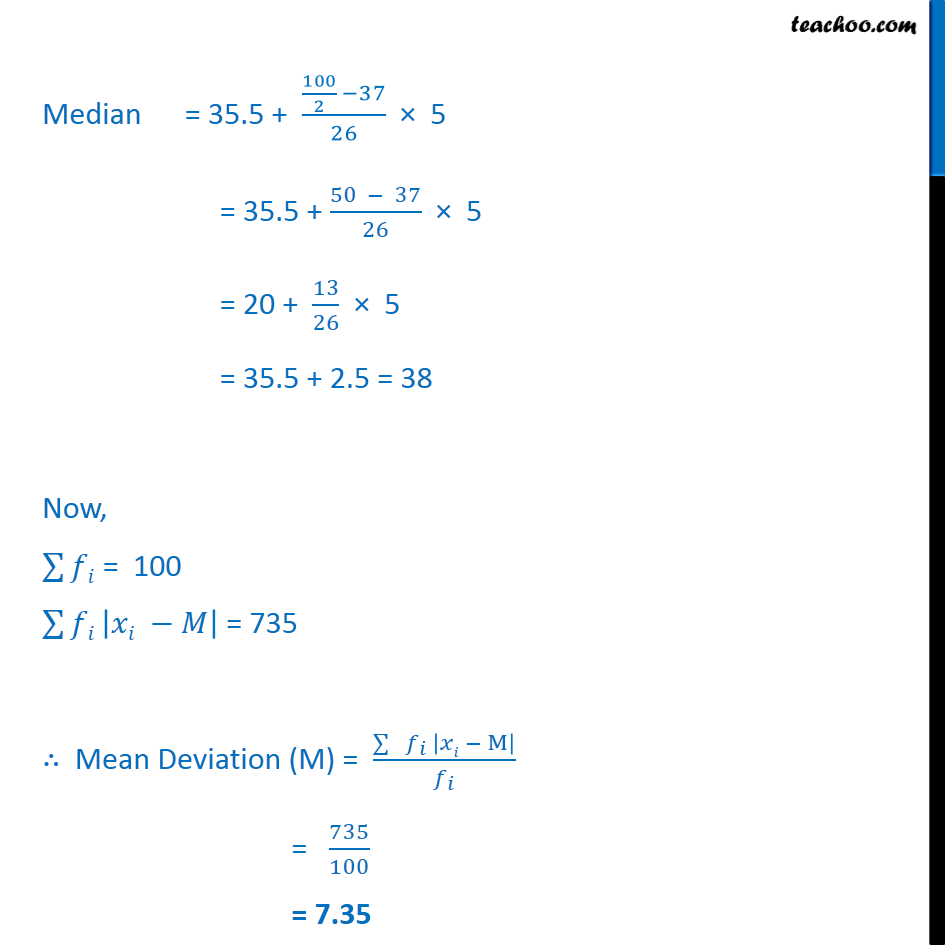Ex 13.1

Chapter 13 Class 11 Statistics
Serial order wiseLearn in your speed, with individual attention - Teachoo Maths 1-on-1 Class

### Transcript

Ex15.1, 12 Calculate the mean deviation about median age for the age distribution of 100 persons given below: Converting the given data in continuous frequency by subtracting 0.5 from lower age limit adding and 0.5 in upper limit N = 128 = 100 Median Class = ( /2)^ term = (100/2)^ term = 50th term In above data, cumulative frequency of class 35.5 40.5 is 63 which is greater than 50. Median class = 35.5 40.5 Median = + ( /2 )/ Where, = lower limits of median class N = sum of frequencies = frequency of median class C = Cumulative frequency of class before median class Here, = 35.5, N = 100, C = 37, = 5, = 26 Median = 35.5 + (100/2 37)/26 5 = 35.5 + (50 37)/26 5 = 20 + 13/26 5 = 35.5 + 2.5 = 38 Now, 128 = 100 128 | | = 735 Mean Deviation (M) = ( _ | M| )/ _ = 735/100 = 7.35